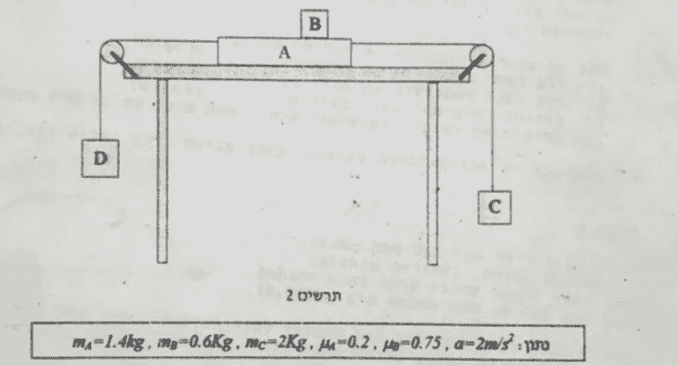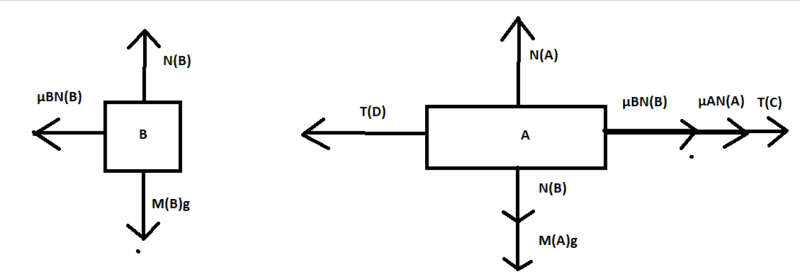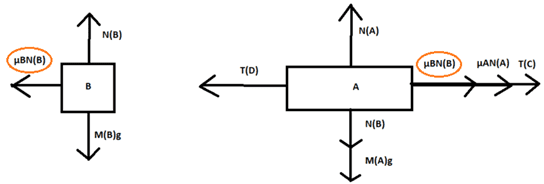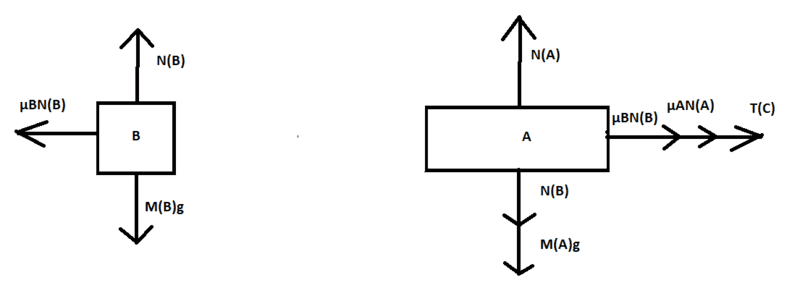# Newton's laws -- Blocks and pulleys problem with friction...

## Homework StatementThe coefficient of friction between A and the table is μA.
The coefficient of friction between A and B is μB.
A, B, C and D all move with an acceleration of 2m/s^2 (A and B move to the left).
Find the force that the friction between the bodies apply on B.
Find the tension of the yarn that connects A and C.
Find the mass of D.
At a certain moment, the yarn which connects A and D is torn. Will A and B still move together? (Show with calculations)
mA=1.4kg ,mB=0.6kg, mC=2kg, μA=0.2, μB=0.75, a=2m/s^2
*Both times the static and dynamic coefficients are equal.

F=ma

## The Attempt at a Solution

The last question is what I am stuck in, but I don't have the answers so I will show my calculations prior to that.
Forces on A and B: (I hope everything is clear)mBg=6N so NB=6N, now we could see that according to ma=F, 1.2=F, so the force is 1.2N.
Now if we look at C we could see that 4=TC-20, TC=24N.
Now if we look at A we could see that: mAg=14N, so NA=20N.
Using ma=F: 2.8=TD-1.2-24-0.2*20 so 2.8=TD-29.2, TD=32N
Looking at D: 2mD=10mD-TD, mD=4kg.

Now I am not sure what to do, I tried to draw the forces again, but from there I don't really know what to do. How can I know if C moves now? If it doesn't then A and B doesn't move, but if it does I don't know how to reach the answer.

#### Attachments

TSny
Homework Helper
Gold Member
In your diagram you have assumed that the friction force between A and B is given by μN.Is this friction force static or dynamic (kinetic)? Can you see why you should not assume that the friction force is μN? Fortunately, in your calculations you never used this assumption. Your results so far look correct to me.

How can I know if C moves now? If it doesn't then A and B doesn't move, but if it does I don't know how to reach the answer.

At the instant the yarn breaks, I think they want you to assume the system is in motion according to the setup of the first part of the problem. So, all the blocks have the same speed at the instant the yarn breaks. You'll need to draw new free body diagrams.

#### Attachments

In your diagram you have assumed that the friction force between A and B is given by μN.
View attachment 217011
Is this friction force static or dynamic (kinetic)? Can you see why you should not assume that the friction force is μN? Fortunately, in you calculations you never used this assumption. Your results so far look correct to me.

At the instant the yarn breaks, I think they want you to assume the system is in motion according to the setup of the first part of the problem. So, all the blocks have the same speed at the instant the yarn breaks. You'll need to draw new free body diagrams.

I can see why I should not assume this, I just used it to draw the diagram but I admit it wasn't clever to do.
I will try what you said, I just never assume anything and due to that it quite often I can't solve problems. I wish those things would be clearer...

scottdave
Homework Helper
If a block is in static condition with the surface (for example block B moves with block A), then the frictional force is enough to keep it in that condition, which may be less than μstaticN. If the amount of force necessary to keep it static (not sliding) is greater than μstaticN, then the block is sliding and the amount of force is equal to μkineticN. Note that the problem statement assigns μkinetic equal to μstatic for each of the surfaces.

I would recommend finding some practice problems where they have the block on an incline and you have to figure out "will is slide" or "how fast does it slide".

haruspex
Homework Helper
Gold Member
2020 Award
Fortunately, in your calculations you never used this assumption.
... And anyway, in this part of the question, using g=10m/s2, it happens that the assumption is correct.
At the instant the yarn breaks, I think they want you to assume the system is in motion
Does that matter? It doesn't affect the accelerations and forces, does it?
Even if the static coefficient at the table were greater than the kinetic, it would have to "pass through" the static condition to reverse direction.

That said, there can be a very subtle snag with these "cut string" questions. Any real string has some elasticity. A proper treatment would allow some string constant k, but having solved for the general case take the limit as k tends to infinity.
A consequence is that the tension in the uncut string does not instantly change. It can only change by virtue of the attached object, newly freed from its other string, yielding to that tension. So to answer the question above strictly, whether the two blocks remain in a static relationship, the unchanged tension in the uncut string should be used.
That is clearly not the intent of the problem setter, though.

Last edited:
TSny
Homework Helper
Gold Member
... And anyway, in this part of the question, using g=10m/s2, it happens that the assumption is correct.
The force of friction on B is 1.2 N. But μBNB = (0.75)(6.0N) = 4.5 N

Does that matter? It doesn't affect the accelerations and forces, does it?
Even if the static coefficient at the table were greater than the kinetic, it would have to "pass through" the static condition to reverse direction.
No, it doesn't matter. Any speed that the blocks have at the instant the yarn snaps does not affect the forces and accelerations. But, I think it's a good part of the exercise for the student to think through this.

That said, there can be a very subtle snag with these "cut string" questions. Any real string has some elasticity. A proper treatment would allow some string constant k, but having solved for the general case take the limit as k tends to infinity.
A consequence is that the tension in the uncut string does not instantly change. It can only change by virtue of the attached object, newly freed from its other string, yielding to that tension. So to answer the question above strictly, whether the two blocks remain in a static relationship, the unchanged tension in the uncut string should be used.
Interesting. Would this affect whether or not the blocks are in a static relationship 1 second, say, after the yarn breaks?

haruspex
Homework Helper
Gold Member
2020 Award
The force of friction on B is 1.2 N. But μBNB = (0.75)(6.0N) = 4.5 N
I should have magnified the diagram. I read the A subscript on μA as a B.
Would this affect whether or not the blocks are in a static relationship 1 second, say, after the yarn breaks?
It depends. If the initially high tension makes it enough to overcome static friction and the asymptotic tension overcomes kinetic but not static, then yes, it will make a long term difference to the outcome.

In your diagram you have assumed that the friction force between A and B is given by μN.
View attachment 217011
Is this friction force static or dynamic (kinetic)? Can you see why you should not assume that the friction force is μN? Fortunately, in your calculations you never used this assumption. Your results so far look correct to me.

At the instant the yarn breaks, I think they want you to assume the system is in motion according to the setup of the first part of the problem. So, all the blocks have the same speed at the instant the yarn breaks. You'll need to draw new free body diagrams.

So, after the yarn is torn I am getting this:So that basically means that they won't move together because their acceleration are different, correct?

#### Attachments

... And anyway, in this part of the question, using g=10m/s2, it happens that the assumption is correct.

Does that matter? It doesn't affect the accelerations and forces, does it?
Even if the static coefficient at the table were greater than the kinetic, it would have to "pass through" the static condition to reverse direction.

That said, there can be a very subtle snag with these "cut string" questions. Any real string has some elasticity. A proper treatment would allow some string constant k, but having solved for the general case take the limit as k tends to infinity.
A consequence is that the tension in the uncut string does not instantly change. It can only change by virtue of the attached object, newly freed from its other string, yielding to that tension. So to answer the question above strictly, whether the two blocks remain in a static relationship, the unchanged tension in the uncut string should be used.
That is clearly not the intent of the problem setter, though.

I don't understand how this can be solved without this assumption. If it doesn't matter how can you know how the forces would look like, and if it would be enough to get A moving?

haruspex
Homework Helper
Gold Member
2020 Award
I don't understand how this can be solved without this assumption. If it doesn't matter how can you know how the forces would look like, and if it would be enough to get A moving?
Just treat it as though mass D were never present. Somebody was holding A in place then let go.

Just treat it as though mass D were never present. Somebody was holding A in place then let go.
From what I understand TSny was correct when he said I should make this assumption because of the way the question is written. If that's the case, are my diagram and explanation above correct?

haruspex
Homework Helper
Gold Member
2020 Award
From what I understand TSny was correct when he said I should make this assumption because of the way the question is written. If that's the case, are my diagram and explanation above correct?
What assumption do you mean?
I am not at all certain what your diagrams in post #8 indicate. Looks to me like you have the two blocks accelerating in opposite directions, but that makes no sense.

What assumption do you mean?
I am not at all certain what your diagrams in post #8 indicate. Looks to me like you have the two blocks accelerating in opposite directions, but that makes no sense.
This: (what TSny wrote)
At the instant the yarn breaks, I think they want you to assume the system is in motion according to the setup of the first part of the problem. So, all the blocks have the same speed at the instant the yarn breaks. You'll need to draw new free body diagrams.
This diagram is supposed to show the forces on each block after the yarn is torn.

haruspex
Homework Helper
Gold Member
2020 Award
This: (what TSny wrote)
At the instant the yarn breaks, I think they want you to assume the system is in motion according to the setup of the first part of the problem. So, all the blocks have the same speed at the instant the yarn breaks. You'll need to draw new free body diagrams.
This diagram is supposed to show the forces on each block after the yarn is torn.
You are perhaps confusing direction of movement with direction of acceleration.
Since A is moving left, with some inertia, you are right that after the cut the friction from the table still acts to the right on A at first. (I was wrong to say the the initial leftward movement was completely irrelevant.) But think carefully about the interaction between A and B.
Before the cut, friction acts to the left on B because A is accelerating leftwards. After the cut, which way does A accelerate? What does that mean for the contact between A and B?

You are perhaps confusing direction of movement with direction of acceleration.
Since A is moving left, with some inertia, you are right that after the cut the friction from the table still acts to the right on A at first. (I was wrong to say the the initial leftward movement was completely irrelevant.) But think carefully about the interaction between A and B.
Before the cut, friction acts to the left on B because A is accelerating leftwards. After the cut, which way does A accelerate? What does that mean for the contact between A and B?
Wait, so the direction of the friction between A and B on each block would be determined by the direction of A's acceleration and not by the direction of its movement?

haruspex
Homework Helper
Gold Member
2020 Award
Wait, so the direction of the friction between A and B on each block would be determined by the direction of A's acceleration and not by the direction of its movement?
It is determined by the relative movement, or tendency to relative movement, of the two surfaces in contact.
Just before the yarn is cut, A and B are moving at the same velocity, but because A is being accelerated left there is friction between the surfaces opposing the tendency for A to move left faster than B. Thus the friction acts to the left on B and to the right on A.
When the yarn has been cut, there is no leftward force on A, except possibly from B. So friction between A and B no longer has to oppose A's tendency to move left faster than B. Indeed, except for B, all the forces on A are now to the right, so the tendency is for A to accelerate to the right. That means it will tend to have a rightward movement relative to B, and the frictional forces between the two reverse.

It is determined by the relative movement, or tendency to relative movement, of the two surfaces in contact.
Just before the yarn is cut, A and B are moving at the same velocity, but because A is being accelerated left there is friction between the surfaces opposing the tendency for A to move left faster than B. Thus the friction acts to the left on B and to the right on A.
When the yarn has been cut, there is no leftward force on A, except possibly from B. So friction between A and B no longer has to oppose A's tendency to move left faster than B. Indeed, except for B, all the forces on A are now to the right, so the tendency is for A to accelerate to the right. That means it will tend to have a rightward movement relative to B, and the frictional forces between the two reverse.
Now I am not actually sure how to calculate if they will be able to move together. I understood how the forces act, and what I did is to assume that they move together and then check if μB is smaller or equal to 0.75. However I am not sure if I am allowed to make this assumption, how would you solve it?

haruspex
•